Published

# Simple light organ

Simple nice 3 color, 4 level LIGHT ORGAN with Arduino Pro Mini

IntermediateShowcase (no instructions)110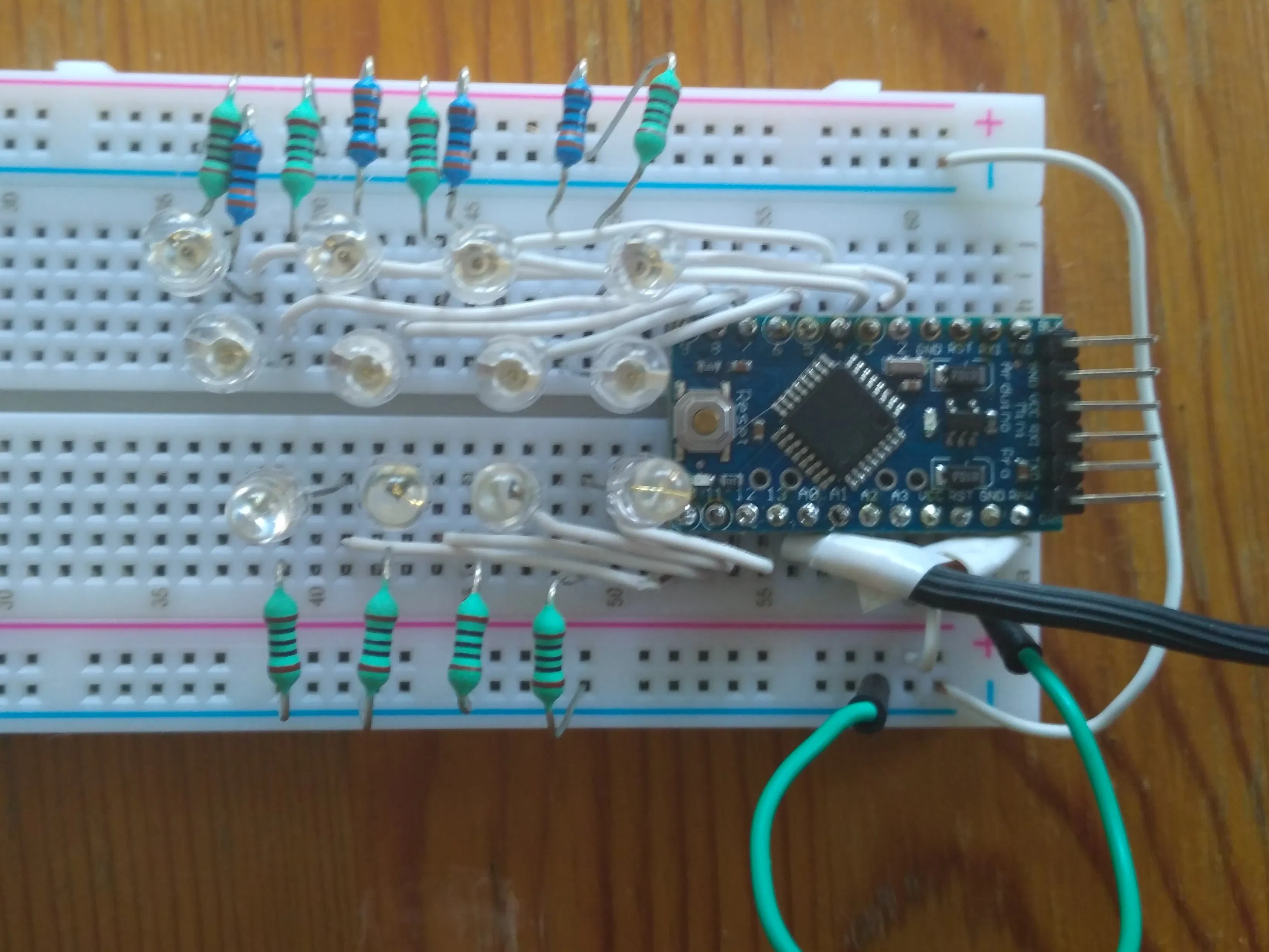## Things used in this project

### Hardware components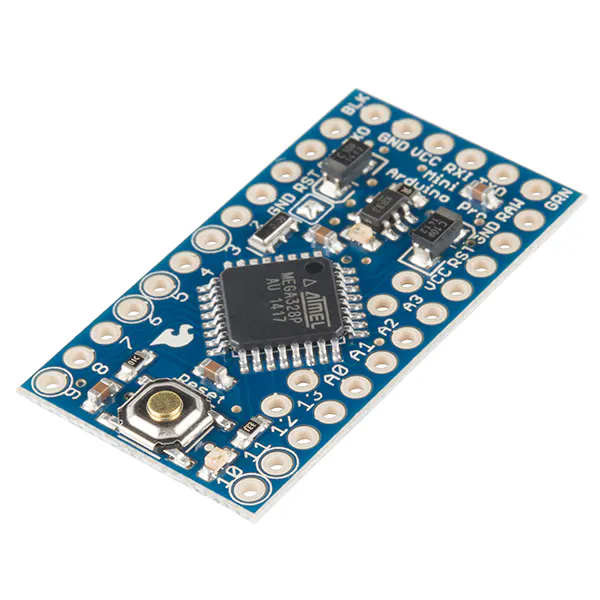SparkFun Arduino Pro Mini 328 - 3.3V/8MHz
×15 mm LED: Red
×4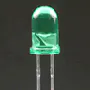5 mm LED: Green
×4LED, Blue
×4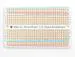×1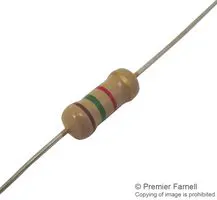Through Hole Resistor, 300 ohm
×12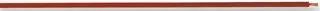Wire, Hook Up
×18

### Software apps and online services

 Scilab I wrote the following Scilab code to calculate filter parameters: // calculating parameter for Exponential moving average cut-off frequency // simple low pass filter from dsp.stackexchange.com/questions/40462/exponential-moving-average-cut-off-frequency Fs = 20000; fthreedb = 80; // 1000 Hz 0.0956 10kHz 0.605 30kHz 0.828 @ Fs 62.5 kHz // 1000 Hz 0.1539 10kHz 0.747 @ Fs 37.5 kHz // 420 Hz 0.0623 1900 Hz 0.251 @ 41 kHz // 500 Hz 0.0737 5kHz 0.5187 @ 41 kHz // 2000 Hz 0.2622 8kHz 0.6655 @ 41 kHz omegathreedb = 2*%pi*fthreedb / Fs; tenek = 2*(1-cos (omegathreedb)); oneok = 2*(cos (omegathreedb) - 1); disp (tenek); disp (oneok); p = [1 tenek oneok]; // masodfoku fuggveny polinom egyutthatoi disp(roots(p)); // masodfoku fuggveny gyokeinek kiirasa x = cos(omegathreedb) - 1 + sqrt(cos(omegathreedb)^2 - 4*cos(omegathreedb) + 3); disp(x) // masodfoku fuggveny megoldokeplete a negyzetgyok allatti tag hozzaadasaval

## Schematics

### light organ

the source code file contains the simple text drawing schematics of the circuit

## Code

### Simple nice 3 color, 4 level LIGHT ORGAN with Arduino Pro Mini

C/C++
```//                               ****************************************************************************
//                               *                                                                          *
//                               *       Simple nice 3 color, 4 level LIGHT ORGAN with Arduino Pro Mini     *
//                               *                           ver 1.0                                        *
//                               *                                                                          *
//                               ****************************************************************************
//
//
//                                       Vcc = 3.3V
//                                             ^
//                                             |
//                                  ___|___|___|___|___|___|___
//                                 |  GND GND VCC RXI TXD DTR  |                         GND
//                               |TXD                     RAW|
//                                 |                           |
//                               |RXI                     GND||
//                                 |                           |
//                               |RST                     RST|
//                                 |                           |
//                               |GND                     VCC|
//          R1=300R___ red LED1    |                           |                           < GND from integrated amplifier's phones output
//     ||___|<||2                        A3|
//          R2=300R___ red LED2    |                           |                           < signal from integrated amplifier's phones output
//     ||___|<||3    Arduino Pro Mini    A2|                                (since no AGC the volume needs to be adjusted to get appropriate signal level so that the LEDs turn on)
//          R3=300R___ red LED3    |                           |
//     ||___|<||4      w/ ATMega328      A1|>  used only for development (signal to oscilloscope to measure sampling freq.)
//          R4=300R___ red LED4    |                           |
//     ||___|<||5       8MHz/ 3.3V       A0|
//          R5=300R___green LED5   |                           | blue LED9       ___ R9= 300R
//     ||___|<||6                        13||>|___||
//          R6=300R___green LED6   |                           | blue LED10      ___ R10=300R
//     ||___|<||7                        12||>|___||
//          R7=300R___green LED7   |                           | blue LED11      ___ R11=300R
//     ||___|<||8                        11||>|___||
//          R8=300R___green LED8   |                           | blue LED12      ___ R12=300R
//     ||___|<||9                        10||>|___||
//                                 |___________________________|
//
//

#ifndef cbi
#define cbi(sfr, bit) (_SFR_BYTE(sfr) &= ~_BV(bit))
#endif
#ifndef sbi
#define sbi(sfr, bit) (_SFR_BYTE(sfr) |= _BV(bit))
#endif
#define bit_is_set(sfr, bit) (_SFR_BYTE(sfr) & _BV(bit))

//Constants and Global Variables

const int stL1 =  20;           // threshold for step1  lowpass out
const int stL2 =  25;           // threshold for step2  lowpass out
const int stL3 =  30;           // threshold for step3  lowpass out
const int stL4 =  40;           // threshold for step4  lowpass out
const int stM1 =  16;           // threshold for step1  bandpass out
const int stM2 =  25;           // threshold for step2  bandpass out
const int stM3 =  32;           // threshold for step3  bandpass out
const int stM4 =  48;           // threshold for step4  bandpass out
const int st1 =  40;            // threshold for step1  highpass out
const int st2 =  55;            // threshold for step2  highpass out
const int st3 =  70;            // threshold for step3  highpass out
const int st4 =  80;            // threshold for step4  highpass out

int samplVal = 0;                 //initialization of sensor variable, equivalent to EMA Y
int EMA_SVL = 0;                  //initialization of EMA_S very low pass
int EMA_SL = 0;                   //initialization of EMA_S low pass
int EMA_SM = 0;                   //initialization of EMA_S middle pass
int EMA_SH = 0;                   //initialization of EMA_S high pass
int lowpass = 0;
int bandpass = 0;
int highpass = 0;

void setup() {

// Turn off PWM timers
cbi(TCCR1A, COM1A1);
cbi(TCCR1A, COM1B1);
cbi(TCCR0A, COM0A1);
cbi(TCCR0A, COM0B1);
cbi(TCCR2A, COM2A1);
cbi(TCCR2A, COM2B1);

// Set the output pins
pinMode(13, OUTPUT);          // Channel_1    low pass  level 1
pinMode(12, OUTPUT);          // Channel_1    low pass  level 2
pinMode(11, OUTPUT);          // Channel_1    low pass  level 3
pinMode(10, OUTPUT);          // Channel_1    low pass  level 4
pinMode(A1, OUTPUT);          // cycle output signal to measure sampling rate neccessary for calculating EMA alphas
pinMode(2, OUTPUT);           // Channel_2    high pass  level 1
pinMode(3, OUTPUT);           // Channel_2    high pass  level 2
pinMode(4, OUTPUT);           // Channel_2    high pass  level 3
pinMode(5, OUTPUT);           // Channel_2    high pass  level 4
pinMode(6, OUTPUT);           // Channel_3    band pass  level 1
pinMode(7, OUTPUT);           // Channel_3    band pass  level 2
pinMode(8, OUTPUT);           // Channel_3    band pass  level 3
pinMode(9, OUTPUT);           // Channel_3    band pass  level 4

//                      https://www.instructables.com/id/Girino-Fast-Arduino-Oscilloscope/
// Set the sample timer's prescaler to 2, enabling max sample freq = 8000KHz/13/2   (13 is the nr of ADC succ.appr. cycles)
// actual sample frequency depends on the code (time of operations between sampling - no interrupts applied) in this case it is 20kHz
// audiophiles should not worry about missing high frequency part, because above 7kHZ there is negligible power - no influence on the lights

EMA_SL = 0;                               //set EMA_S. for t=1
EMA_SVL = 0;
EMA_SH = 0;
}

void loop() {

// Simple digital filters applied with  exponential moving average (EMA) algorythm:
// https://dsp.stackexchange.com/questions/40462/exponential-moving-average-cut-off-frequency
// f3db filter frequencies (and the connected EMA alpha coefficients) are selected so that EMA algorythm can be calculated with
// simple fixed operations (add, sub, bit shift: division by 2,4,8,16,32) to keep speed, float operations avoided

EMA_SVL =  (samplVal / 32) + EMA_SVL - (EMA_SVL / 32);        // EMA alpha very low = 1/32  -> f3db = 102 Hz @ Fs=20kHz
EMA_SL = (samplVal / 16) + EMA_SL - (EMA_SL / 16);            // EMA alpha low = 1/16  -> f3db = 315 Hz @ Fs=20kHz
EMA_SM = (samplVal / 8) + (samplVal / 32) + EMA_SM - (EMA_SM / 8)- (EMA_SM / 32);     // EMA alpha middle  = 5/32 ->  f3db = 540 Hz @ Fs=20kHz
EMA_SH = (samplVal / 2) + (samplVal / 8)+ EMA_SH - (EMA_SH / 2) - (EMA_SH / 8);       // EMA alpha high = 5/8 ->  f3db = 3400 Hz @ Fs=20kHz
lowpass = EMA_SL - EMA_SVL;
bandpass = EMA_SM - EMA_SL;
highpass = EMA_SH - EMA_SM;
// PORTB &= B11000000;                       // turn off all LEDs
// PORTD &= B00000011;
digitalWriteB_za (abs(lowpass));          // turn on Channel_1 LEDs according to lowpass value calculated with the latest sample value
digitalWriteBD_za (abs(bandpass));        // turn on Channel_3 LEDs according to bandbass value calculated with the latest sample value
digitalWriteD_za (abs(highpass));         // turn on Channel_2 LEDs according to highpass value calculated with the latest sample value

}

//  the code in he following link has been modified according to this application's requiremets

{
uint8_t sample_val;                    // sampled analog value
uint8_t tempPC;                        // temporary value of PORT C

// set the analog reference (high two bits of ADMUX: REFS1 = 0, REFS0 = 1 ->  ADC Vref = 3.3V)

// we have to read only ADCH ,  due to limited accuracy the 2 bits in ADCL are not needed

tempPC  = PORTC ^ B00000010;           // Invert cycle output signal
PORTC = tempPC;                        // Set A1 output which enables to measure sampling rate neccessary for calculating EMA alphas
return sample_val;
}

// 3 digital write functions per 3 color channels
// https://www.arduino.cc/en/Reference/PortManipulation

void digitalWriteB_za (int val)
{
uint8_t oldSREG = SREG;
uint8_t tempPB;                    // temporary value of PORTB
cli();

if (val < stL1) {
PORTB &= B11000011;                                     // all Channel_1 LEDs off
} else {
tempPB  = PORTB & B11000011;
if (val < stL2) {
PORTB = tempPB | B00100000;                      // set pin13 = PB5 to HIGH
} else {
if (val < stL3) {
PORTB = tempPB | B00110000;                    // set pin13 = PB5, pin12 = PB4 to HIGH
} else {
if (val <  stL4) {
PORTB  = tempPB | B00111000;         // set pin13 = PB5, pin12 = BB4, pin11 = PB3 to HIGH
} else {
PORTB = tempPB | B00111100;           // all Channel_1 LEDs on
}
}
}
}
}

void digitalWriteD_za (int val)
{
uint8_t oldSREG = SREG;
uint8_t tempPD;               // temporary value of PORTD
cli();

if (val < st1) {
PORTD &= B11000011;                                     // all Channel_2 LEDs off
} else {
tempPD  = PORTD & B11000011;
if (val < st2) {
PORTD = tempPD | B00000100;                      // set pin2 = PD2 to HIGH
} else {
if (val < st3) {
PORTD = tempPD | B00001100;                    // set pin2 = PD2, pin3 = PD3 to HIGH
} else {
if (val <  st4) {
PORTD  = tempPD | B00011100;         // set pin2 = PD2, pin3 = PD3, pin4 = PD4 to HIGH
} else {
PORTD = tempPD | B00111100;           // all Channel_2 LEDs on
}
}
}
}
SREG = oldSREG;
}

void digitalWriteBD_za (int val)
{
uint8_t oldSREG = SREG;
uint8_t tempPD;               // temporary value of PORTD
uint8_t tempPB;               // temporary value of PORTB
cli();

if (val < stM1) {
PORTD &= B00111111;                                  // all Channel_3 LEDS off
PORTB &= B11111100;
} else {
tempPD = PORTD & B00111111;
tempPB = PORTB & B11111100;
if (val < stM2) {
PORTD = tempPD | B01000000;                   // set pin6 = PD6 to HIGH
PORTB = tempPB;
} else {
if (val < stM3) {
PORTD = tempPD | B11000000;
PORTB = tempPB;
} else {
if (val <  stM4) {
PORTD = tempPD | B11000000;
PORTB = tempPB | B00000001;
} else {
PORTD = tempPD | B11000000;
PORTB = tempPB | B00000011;      // all Channel_3 LEDs on
}
}
}
}
SREG = oldSREG;
}
```

## Credits

### aranyzs

1 project • 0 followers